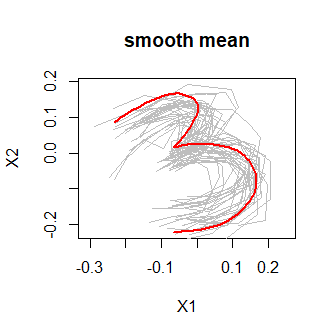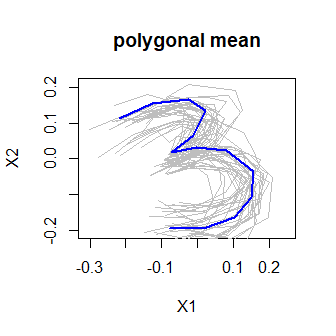# How to estimate elastic shape means with elastes

This vignette describes basic usage of elastes in R.

## Installation

Like many other R packages, the simplest way to obtain elastes is to install it directly from CRAN. Type the following command in your R console:

install.packages("elastes")

## Quick Start

The purpose of this section is to give users a general sense of the package. We will briefly go over the main functions, basic operations and outputs

Firstly, load up the elastes package.

library(elastes)

We load a set of 30 sparse handwritten digit 3’s from the shapes package for illustration. You may have to install the shapes package first.

# install.packages("shapes")
library(shapes)
data(digit3.dat)

The data still has the form of a three dimensional array. We will have to convert it to a list of data.frames, where each data.frame corresponds to one curve.

digit3 <- apply(digit3.dat, MARGIN = 3, FUN = function(curve){
data.frame(X1 = curve[,1], X2 = curve[,2])
})

We can compute a functional mean curve by calling compute_elastic_shape_mean by specifiying the set of knots to be used and the type of mean. Here we estimate and plot a smooth mean using 11 equidistant knots. The call to plot.elastic_shape_mean shows the estimated mean function, together with the so called Procrustes fits - the centered and rotation plus scaling aligned data curves.

mean_smooth <- compute_elastic_shape_mean(
digit3,
knots = seq(0, 1, length = 11),
type = "smooth"
)
plot(mean_smooth, main = "smooth mean")Here we estimate and plot a polygonal mean using 13 equidistant knots.

mean_poly <- compute_elastic_shape_mean(
digit3,
knots = seq(0, 1, length = 13),
type = "polygon"
)
plot(mean_poly, col = "blue", main = "polygonal mean")The estimated mean is the leading eigenfunction of the smoothed Hermitian covariance surface of the data curves. In the covariance estimation, the used penalty can be controlled by the penalty parameter.
The method can control for different types of measurement-error variance along t, by setting the var_type parameter.
help(compute_elastic_shape_mean)